# Seedlings

The meadow was divided into 30 equally large parts and then it was calculated how many seedlings are in each part. It was found that there were no seedlings in six parts, five parts contained one seedling, twelve parts two seedlings and seven parts three seedlings. Assess whether the seedlings are randomly distributed in the meadow.

Result

x=

### Step-by-step explanation:Did you find an error or inaccuracy? Feel free to write us. Thank you!Tips to related online calculators
Looking for help with calculating arithmetic mean?
Looking for a statistical calculator?

#### You need to know the following knowledge to solve this word math problem:

We encourage you to watch this tutorial video on this math problem:

## Related math problems and questions:

• Poisson distribution - daisies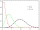The meadow behind FLD was divided into 100 equally large parts. Subsequently, it was found that there were no daisies in ten of these parts. Estimate the total number of daisies in the meadow. Assume that daisies are randomly distributed in the meadow.
• Lesson exercising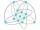The lesson of physical education, pupils are first divided into three groups so that each has the same number. The they redistributed, but into six groups. And again, it was the same number of children in each group. Finally they divided into nine equal g
• The average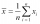The average of one set of 4 numbers is 35. The average of another set of number is 20. The average of the numbers in the two sets is 30. How many numbers are there in the other set?
• Certificate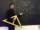There is 31 students in a class. From mathematics was'nt worse mark than 2. Average mark in mathematics was 2. How many students have mark 1 and how many mark 2?On the meadow grazing horses, cows, and sheep, together with less than 200. If cows were 45 times more, horses 60 times more, and sheep 35 times more than there are now, their numbers would equally. How many horses, cows, and sheep are on the meadow toget
• CarpentersCarpenters 1 and 2 spend ten days and five days respectively to make one table. If 50 tables were made by the first carpenter and 30 tables were made by the second carpenter, What is the average time spent on the products?
• Controller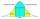Output Controller of the company in the control of 50 randomly selected products found that 37 of them had no defects, 8 has only one flaw, three had two defects, and two products had three defects. Determine the standard deviation and coefficient of vari
• Range, mean, medianAges of 7 employees in an office are given below 32, 42, 30, 32, 33, 23, 32 Find a) Range b) Mean c) Median
• Salat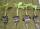Grandmother planted salad. In each row, he planted 13 seedlings. After a morning frost, many seedlings died. In the first row, five seedlings died. In the second row, two seedlings died more than 1st row. In the third row, three seedlings died less than 1
• Final examThere are 5 learners in a class that has written a final exam Aleta scored 55% vera scored 36% and Sibusiso scored 88% if Thoko scored 71% and the class average was 63%. What was Davids score as a percentage?
• Target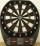Peter, Martin and Jirka were fire in a special target, which had only three fields with values of 12, 18 and 30 points. All boys were firing with the same number of arrows and all the arrows hit the target, and the results of every two boys differed in on
• The mowers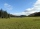The mowers were to mow two meadows, one twice as big as the other. In the first half of the day, they divided into two equal groups. One continued to mow a larger meadow and cut it all by the end of the day. The second group mowed a smaller meadow but did
• Four families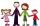Four families were on a joint trip. In the first family, there were three siblings, namely Alica, Betka and Cyril. In the second family were four siblings, namely David, Erik, Filip and Gabika. In the third family, there were two siblings, namely Hugo and
• Jolly gobs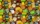Each package of jolly gobs has 72 gobs. If one fourth of the gobs are red and the rest are blue, into how many parts was the group divided? How many parts are red?
• NormThree workers planted 3555 seedlings of tomatoes in one dey. First worked at the standard norm, the second planted 120 seedlings more and the third 135 seedlings more than the first worker. How many seedlings were standard norm?
• Variance and average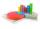Of the 40 values were calculated average mx = 7.5 and variance sx = 2.25. After the control was found to lack the two items of the values of x41 = 3.8 and x42=7. Correct the above characteristics (mx and sx).
• GroupA group of kids wanted to ride. When the children were divided into groups of 3 children, one remain. When divided into groups of 4 children, 1 remains. When divided into groups of 6 children, 1 remains. After divided into groups of 5 children, no one lef